Module 8 - Answers Lesson 1 Answer 1 8.1.1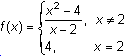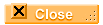Lesson 2 Answer 1 8.2.1 The discontinuity is called an infinite discontinuity because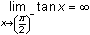and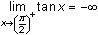.Lesson 3 Answer 1 8.3.1 The value of the function at x = 2 is given by 2(2) + 1 =5. Therefore, the second piece of the function should approach 5 as x approaches 2.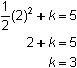Letting k = 3 will make the function continuous at x = 2.Answer 2 8.3.2 The second piece would be shifted up, creating a jump discontinuity.Answer 3 8.3.3 The left hand limit is 5. The right hand limit is 9.Self Test Answer 1 II and III Answer 2 A removable discontinuity (a hole) exists and the hole can be seen in a zoom decimal window at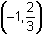.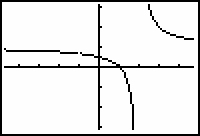Answer 3 k = 0 Answer 4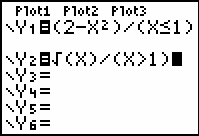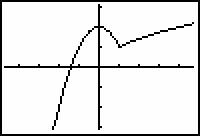Answer 5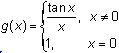©Copyright 2007 All rights reserved. | Trademarks | Privacy Policy | Link Policy